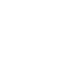# Question of the Day: June 3

If you are reading this in an email you received from me, do not click the link to sat.collegeboard.org below. Use the link to my website that is farther down on the email. If you are seeing this in my blog, dco the SAT Question of the Day by clicking on this link:

http://sat.collegeboard.org/practice/sat-question-of-the-day?src=R&questionId=20130603 (This link takes you to today’s question. If you use my archive, you will see the question related to my SAT explanation for that date.)

The answer is C.  As always, the SAT question is easily solved by thinking about and using my mantra:

• What did the test writers tell me and
• What do I know because they told me that?

All math questions can be solved by approaching them with these questions in mind.  Let’s use it.

For the first triangle, I’m told it is an isosceles right triangle.  (The two base angles are equal).  That means x has to be 45 degrees.  The other triangle is an equilateral triangle (3 equal angles); so, y has to be 60 degrees.  y – x is 15 and 3(15) is 45.

Keep in mind that with the exception of some charts and graph data, the test writers never give you math information you don’t need.  That means that if you are stuck, you must figure out how ALL of the information they give you is useful in answering each question.   Deriving the information you are not given is the key to success on the math questions.

Let’s see what we can do to demystify the ACT.

http://www.act.org/qotd/ (The ACT staff does not put a date on their questions so if you click on an archived blog, you’ll get today’s question and the old explanation. Sorry. The SAT staff has dated their questions; so, the archive is helpful. The ACT folks simply don’t do that.)

Perfect–we have a math question!  Let’s use my math mantra.  (Refer to the SAT explanation above.)

The answer is H.  Did you recognize this question is about the difference of two squares?  That’s what they told you.  x2   is one square and 15 is the other one.  You may have missed seeing this since 15 isn’t a “perfect square” like 16 would have been.  However, every positive number is the square of some number squared!  In this case it is the square root of 15 and don’t forget there is always a positive and negative root.  (Take a look at the ACT explanation and you’ll see all the math steps if you are having trouble with the algebra.  I can’t make all the fancy radical signs, etc. with my website word processor.)  In short, they told you the equation is a perfect square and what you know is there’s always a positive and negative root for a number (15).  That leads you to having two solutions for the equation: a positive one and a negative one.

Have a great week.

The Wizard